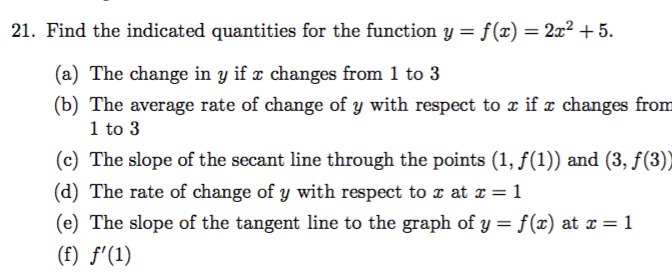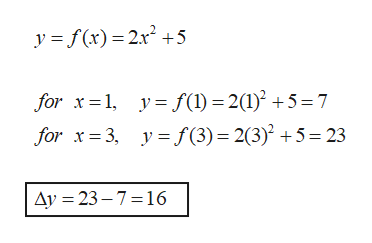# 21. Find the indicated quantities for the function y = f(x)= 2x2 +5(a) The change in y if r changes from 1 to 3(b) The average rate of change of y with respect to x if e changes from1 to 3(c) The slope of the secant line through the points (1, f(1)) and (3, f(3))(d) The rate of change of y with respect to x at x =1(e) The slope of the tangent line to the graph of y = f(x) at x 1(f) f'(1)

Questionhelp_outlineImage Transcriptionclose21. Find the indicated quantities for the function y = f(x)= 2x2 +5 (a) The change in y if r changes from 1 to 3 (b) The average rate of change of y with respect to x if e changes from 1 to 3 (c) The slope of the secant line through the points (1, f(1)) and (3, f(3)) (d) The rate of change of y with respect to x at x =1 (e) The slope of the tangent line to the graph of y = f(x) at x 1 (f) f'(1) fullscreen
check_circle

Step 1

Hi, as per our norms, we answer three sub parts to a question. Since your question has six sub parts, we will answer the first three parts. You may post a separate question to ask the remaining sub parts.

Step 2

The change in y:

Substitute the values of x and get the corresponding y. Calculate the difference in values of y to know the change.help_outlineImage Transcriptionclosey f(x) 2x2+5 for x 1 y f(1) = 2(1)2 +5= 7 for x3, y f(3) 2(3) 5=23 Ay 23-7 16 fullscreen
Step 3

Average rate of change of y...

### Want to see the full answer?

See Solution

#### Want to see this answer and more?

Solutions are written by subject experts who are available 24/7. Questions are typically answered within 1 hour.*

See Solution
*Response times may vary by subject and question.
Tagged in

### Functions